# HelloKoding

Practical coding guides

# Graph Data Structure

A graph is a data structure used for organizing an interconnected network. It is a non-linear data structure consisting of a collection of vertices (nodes) and edges

• A vertex represents an entity (object) (for example, student)
• An edge is a line or arc that connects a pair of vertices in the graph, represents the relationship between entities

## Examples

• A computer network is a graph with computers are vertices and network connections between them are edges
• The World Wide Web is a graph with web pages are vertices and hyperlinks are edges

## Types of Graphs

### Undirected vs Directed graph

• An undirected graph has all edges that don’t have a direction, each edge can be travel in both directions (two-way relationships)
• A directed graph has all edges that have a direction, each edge can be travel only in a single direction (one-way relationship)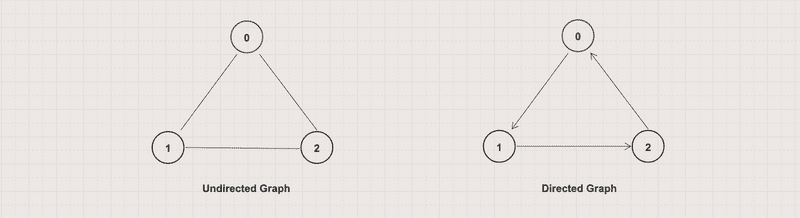### Cyclic vs Acyclic graph

• A cyclic graph has at least a cycle (existing a path from at least one node back to itself)
• An acyclic graph has no cycles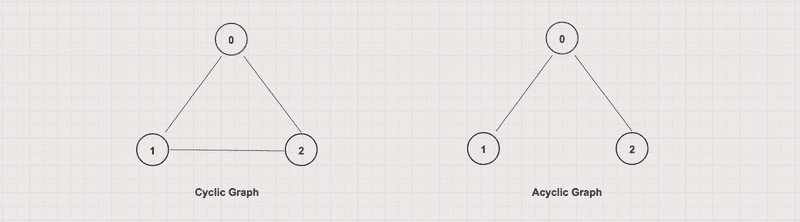### Connected vs Disconnected graph

• A connected graph has no unreachable vertices (existing a path between every pair of vertices)
• A disconnected graph has at least an unreachable vertex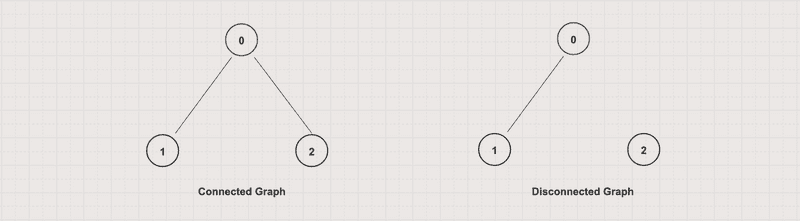### Tree vs Forrest

• A tree is a connected acyclic undirected graph
• A forest is a graph with each connected component a tree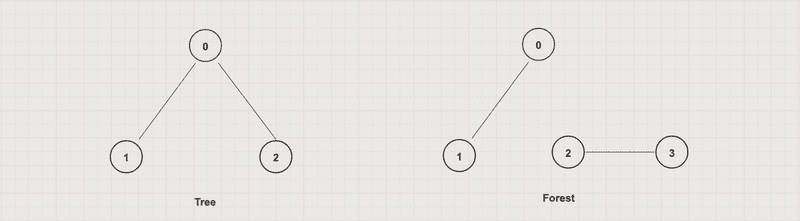### Weighted vs Unweighted graph

• A weighted graph has a weight attached to each edge (for example, the distance between two vertices)
• An unweighted graph has no weight attached to each edge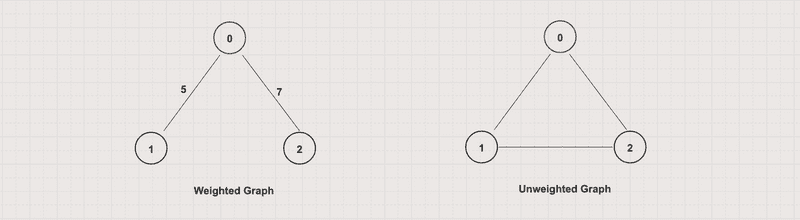## Vertex and Graph degree

Verex degree, denoted as deg(v), is a number of edges connected to the vertex. Graph degree, denoted as Δ(G), is the highest vertex degree in a graph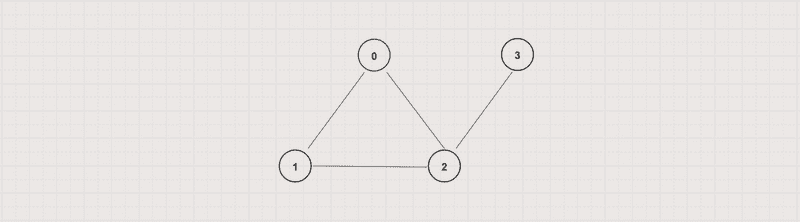In the above graph

• deg(0) = 2, deg(1) = 2, deg(2) = 3, and deg(3) = 1
• Δ(G) = 3

## Vertex Indegree vs Outdegree

In a directed graph, vertex has an indegree and outdegree

• An indegree, denoted as `deg+(v)`, is a number of edges coming to the vertex
• An outdegree, denoted as `deg-(v)`, is a number of edges leaving the vertex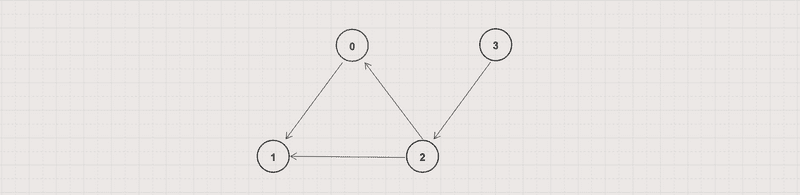In the above graph

• deg+(0) = 1, deg-(0) = 1
• deg+(1) = 2, deg-(1) = 0
• deg+(2) = 1, deg-(2) = 2
• deg+(3) = 0, deg-(3) = 1

## Graph Representations

There are several ways to represent a graph including the Edge list, Adjacency list, and Adjacency matrix. Let take this graph as an example• An edge list is a list or array of all edges where each edge is represented by an array of two vertices

In Java, an edge list can be represented by

``````int[][] graph = {
{0, 1},
{0, 2},
{1, 2},
{2, 3}
};``````
• An adjacency list is a list or array where index represents a vertex and value represents a list of that vertex’s adjacents

In Java, an adjacency list can be represented by

``````int[][] graph = {
{1, 2},
{0, 2},
{0, 1, 3},
{2}
};``````
• An adjacency matrix is a matrix of 0s and 1s indicating the connection between two vertices in which the rows represent source vertices and columns represent destination vertices

In Java, an adjacency matrix can be represented by

``````int[][] graph = {
{0, 1, 1, 0},
{1, 0, 1, 0},
{1, 1, 0, 1},
{0, 0, 1, 0}
};``````

## Basic Graph operations

• Add edge: add an edge between two vertices of the graph
• Traversal: travel each vertex in the graph by using Depth-Frist and Breath-First Search

## Graphs in Java

Java doesn’t have a default Graph implementation. However, you can use other supporting data structures to implement it

## Undirected vs Directed Graph implementation example

• Undirected graph implementation example with an adjacency list

``````package com.hellokoding.datastructure.graph;

import java.util.ArrayList;
import java.util.List;

private int V;

this.V = V;

for (int i = 0; i < V; i++) {
}
}

public Integer getV() {
return this.V;
}

}

public void addEdge(int source, int dest) {
}

for (int i = 0; i < V; i++) {
System.out.printf("Adjacency list of vertex %d is %s %s", i,
}
}

public static void main(String[] args) {
}
}
``````
• Directed graph implementation example with an adjacency list

``````package com.hellokoding.datastructure.graph;

import java.util.ArrayList;
import java.util.List;

private int V;

this.V = V;

for (int i = 0; i < V; i++) {
}
}

public Integer getV() {
return this.V;
}

}

public void addEdge(int source, int dest) {
}

for (int i = 0; i < V; i++) {
System.out.printf("Adjacency list of vertex %d is %s %s", i,
}
}

public static void main(String[] args) {
}
}
``````
• The only difference between the implementations is the `addEdge(source, dest)` method. `addEdge` in an undirected graph adds two-ways direction while in a directed graph adds one-way direction
``````// addEdge in a directed graph
public void addEdge(int source, int dest) {
}

// addEdge in an undirected graph
public void addEdge(int source, int dest) {
}``````

## Weighted Graph implementation example

• Weighted graph implementation example with an adjacency list

``````package com.hellokoding.datastructure.graph;

import java.util.ArrayList;
import java.util.List;

private int V;

this.V = V;

for (int i = 0; i < V; i++) {
}
}

public Integer getV() {
return this.V;
}

}

public void addEdge(int source, int dest, int weight) {
}

for (int i = 0; i < V; i++) {
System.out.printf("Adjacency list of vertex %d is %s %s", i,
}
}

static class WeightedVertex {
final Integer vertex, weight;

public WeightedVertex(int vertex, int weight) {
this.vertex = vertex;
this.weight = weight;
}

public int getWeight() {
return this.weight;
}

public String toString() {
return String.format("%d (weight %d)", vertex, weight);
}
}

public static void main(String[] args) {
}
}
``````

Depth-First Search (DFS) and Breadth-First Search (BFS) are algorithms for traversing or searching a graph

• DFS starts at an arbitrary node and explores as far as possible along each branch before backtracking

BFS starts at an arbitrary node and explores all of the neighbor nodes at the current level before moving on to the next level

Thus DFS is better when the target is far from the source while BFS is better when the target is close to the source

• DFS uses a Stack while BFS uses a Queue data structure to backtrack vertices on the traversal path
• DFS consumes less memory than BFS as BFS has to store all the children each level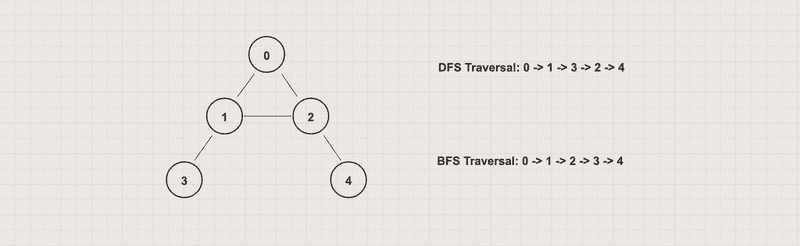## Detect Cycle in a Directed Graph

You can use DFS to detect a cycle in a directed graph. When visiting a vertex v and its adjacency vertices, if there is a back edge (w, v) which directs to v then that graph has a cycle

## Topological Sort with Directed Acyclic Graph

Topological sort is an algorithm which takes a directed acyclic graph and returns a list of vertices in the linear ordering where each vertex has to precede all vertices it directs to

DFS and the above vertex degree theory can be used to implement the topological sort